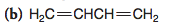Problem: Draw a three-dimensional orbital representation for each of the following molecules, indicate whether each bond in it is a σ or π  bond, and provide the hybridization for each non-hydrogen atom.

FREE Expert Solution
84% (478 ratings)
Problem Details

Draw a three-dimensional orbital representation for each of the following molecules, indicate whether each bond in it is a σ or π  bond, and provide the hybridization for each non-hydrogen atom.What scientific concept do you need to know in order to solve this problem?

Our tutors have indicated that to solve this problem you will need to apply the 3D Hybrid Orbital Drawings concept. If you need more 3D Hybrid Orbital Drawings practice, you can also practice 3D Hybrid Orbital Drawings practice problems .

What is the difficulty of this problem?

Our tutors rated the difficulty of Draw a three-dimensional orbital representation for each of ... as low difficulty.

How long does this problem take to solve?

Our expert Organic tutor, Jonathan took 3 minutes to solve this problem. You can follow their steps in the video explanation above.

What professor is this problem relevant for?

Based on our data, we think this problem is relevant for Professor Maxwell's class at UCF.

What textbook is this problem found in?

Our data indicates that this problem or a close variation was asked in . You can also practice practice problems .